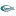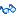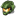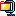Computer Graphics

Above, raytraced pics with Deian Tabakov. Unfortunately, the code is lost. I made a new raytracer.

Even more ancient: Fractals in PowerBASIC.

The woods are lovely, dark, and deep,
But I have promises to keep,
And miles to go before I sleep,
And miles to go before I sleep.
Robert FrostSingle Image Stereogram

The algorithm is adapted from Real-Time Stereograms of the book 'GPU Gems'.

function bmp=stereogram(depth)
% creates greyscale single-image-stereogram of depth field
%  depth     (n,m)-matrix
% which has values in the interval [0,1]
% please scale depth so that an entry with value
%  0 is farthest
%  1 is closest
% the algorithm works best if
%  m ranges between 480 and 1280

ofs=80;
sca=16;

bmp=repmat(uint8(rand(size(depth)+[0 ofs])*255),[1 1 3]);
for cy=1:size(depth,1)
acc=0;
for cx=1:size(depth,2)
inc=depth(cy,cx)*sca+acc;
res=floor(inc);
acc=inc-res;
bmp(cy,ofs+cx,:)=bmp(cy,cx+res,:);
end
end
bmp(:,1:ofs,:)=[];
imshow(bmp)

The use of random noise texture is suitable for animations (hope for loss-less compression)...

If you have a problem,
and you can't solve it alone,
evoke it.
African proverbEdge-Aware Filtering

Eduardo Gastal and Manuel Oliveira have introduced a simple and efficient algorithm to carry out edge-aware image transformations, for instance edge-preserving smoothing. Their method Domain Transform for Edge-Aware Image and Video Processing was published at Siggraph 2011.

Douglas R. Lanman shares source code on Bilateral Filtering. The package also contains a nice cartoon renderer. I have replaced his implementation of the edge-preserving filter with the new, faster algorithm, to speed up the cartoon renderering.

 Edge-Aware Filtering (Matlab) *edgeaware.zip 270 kB
cartoon.m by Douglas R. Lanman, colorspace.m by Pascal Getreuer.

Below is the 40 lines listing of my implementation in Matlab. The emphasis is on simplicity and compactness:

function img=edgeawared(img) % img has double values between 0 and 1

for wid=[3 1]; % support of filter
for c1=1:size(img,1)
dom=ji1domain(img(c1,:,:));
for c3=1:size(img,3)
img(c1,:,c3)=ji1edgeaware(wid,img(c1,:,c3),dom);
end
end
for c1=1:size(img,2)
dom=ji1domain(img(:,c1,:));
for c3=1:size(img,3)
img(:,c1,c3)=ji1edgeaware(wid,img(:,c1,c3)',dom)';
end
end
end

function dom=ji1domain(sig)
sz=size(sig);
sz(find(sz==1))=[];
sig=reshape(sig,sz)';
dom=cumsum(1+sum(abs([zeros(sz(2),1) diff(sig,1,2)]),1));

function res=ji1edgeaware(w,sig,dom)
if nargin==2
dom=cumsum(1+[0 abs(diff(sig))]);
end
dom(end)=ceil(dom(end)); % small error happens here
smp=1:dom(end);
itr=interp1(dom,sig,smp);
res=interp1(smp,ji1filter(w,itr),dom);

function res=ji1filter(w,sig)
w=2*w+1;
x=linspace(-2,2,w);
fil=sqrt(1/pi)*exp(-x.*x);
fil=fil/sum(fil);
num=numel(sig);
f_sig=fft(sig);
f_fil=fft(fil,num);
res=ifft(f_sig.*f_fil);
res=real(res);
res=res([(w+1)/2:end 1:(w-1)/2]);

The figure below illustrates how the algorithm operates on a 1-dimensional domain. The signal is warped onto a streched domain and resampled uniformly. This allows to apply a standard gaussion smoothing filter. Inverting the warp and resampling over the original domain yields the smoothed signal with edges preserved.The extraordinary is in what we do,
not who we are.
Lara Croft